# Structural Engineering - Online Test

Q1. Two beams PQ (fixed at P and with a roller support at Q, as shown in Figure I, which allows vertical movement) and XZ (with a hinge at Y) are shown in the Figures I and II respectively. The spans of PQ and XZ are L and 2L respectively. Both the beams are under the action of uniformly distributed load (W) and have the same flexural stiffness, EI (where, E and I respectively denote modulus of elasticity and moment of inertia about axis of bending). Let the maximum deflection and maximum rotation be δmax1 and θmax1, respectively, in the case of beam PQ and the corresponding quantities for the beam XZ be δmax2 and θmax2, respectively.Which one of the following relationships is true?
Explaination / Solution:
No Explaination.

Q2.  A plane truss with applied loads is shown in the figure.The members which do not carry any force are

Explaination / Solution:

Conditions for zero force members are (i) The member meets at a joint and they are non-collinear and no external force acts at that joint. Both the members will be the zero force members. (ii) When the members meet at joint and two are collinear and no external force acts at the joint then third member will be zero force member. According to the above statements We can say that FT, TG, HU, MP and PL members are zero force members.

Q3.  A rigid member ACB is shown in the figure. The member is supported at A and B by pinned and guided roller supports, respectively. A force P acts at C as shown. Let RAh and RBh be the horizontal reactions at supports A and B, respectively, and RAv be the vertical reaction at support A. Self- weight of the member may be ignored. Which one of the following sets gives the correct magnitudes of RAv, RBh and RAh?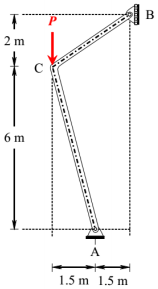Explaination / Solution:
No Explaination.

Q4. A reinforced concrete (RC) beam with width of 250 mm and effective depth of 400 mm is reinforced with Fe415 steel. As per the provisions of IS 456-2000, the minimum and maximum amount of tensile reinforcement (expressed in mm2) for the section are, respectively
Explaination / Solution:

Width of beam (b) = 250mm
Effective depth (d) =400 mm
As per IS-456:200
From clause 26.5.1.1 (a)
Minimum tension reinforcementFrom clause 26.5.1.2(b)
Maximum tension reinforcement = 0.04bd = 0.04 × 250 × 400 = 4000mm2

Q5.  A propped cantilever of span L carries a vertical concentrated load at the mid-span. If the plastic moment capacity of the section is MP, the magnitude of the collapse load isExplaination / Solution: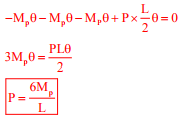Q6. Two plates are connected by fillet welds of size 10 mm and subjected to tension, as shown in the figure. The thickness of each plate is 12 mm. The yield stress and the ultimate tensile stress of steel are 250 MPa and 410 MPa, respectively. The welding is done in the workshop (γmw = 1.25).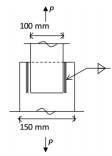As per the Limit State Method of IS 800: 2007, the minimum length (rounded off to the nearest higher multiple of 5 mm) of each weld to transmit a force P equal to 270 kN (factored) is
Explaination / Solution:

Maximum force carried by plates,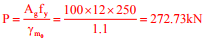Load carried by each weld = P/2 = 136.36kN
For minimum length of weld,
Strength of weld=Load carried by weld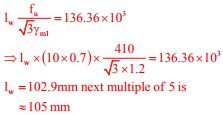Q7. A homogeneous gravity retaining wall supporting a cohesionless backfill is shown in the figure. The lateral active earth pressure at the bottom of the wall is 40 kPa.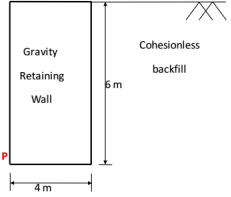The minimum weight of the wall (expressed in kN per m length) required to prevent it from overturning about its toe (Point P) is
Explaination / Solution: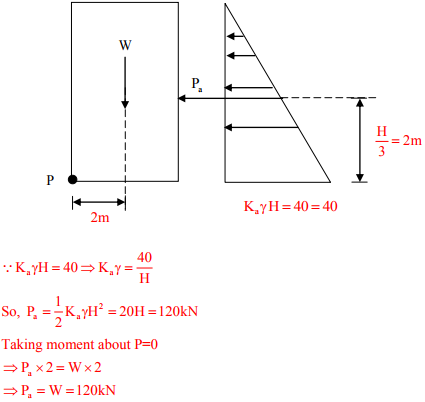Q8. A column of size 450 mm × 600 mm has unsupported length of 3.0 m and is braced against side sway in both directions. According to IS 456:2000, the minimum eccentricities (in mm) with respect to major and minor principle axes are
Explaination / Solution:Q9. A guided support as shown in the figure below is represented by three springs (horizontal, vertical and rotational) with stiffness kx, ky and kθ respectively. The limiting values of kx, ky and kθ are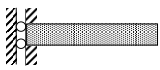Explaination / Solution:

As rotation and horizontal deflection in zero as per given figure. Therefore its stiffness is '∞' as deflection = 0. stiffness= Force/deflection and stiffness is zero in y direction

Q10. A steel member ‘M’ has reversal of stress due to live loads, whereas another member ‘N’ has reversal of stress due to wind load. As per IS 800:2007, the maximum slenderness ratio permitted is
Explaination / Solution:

M – due to live load
N – due to wind load
As per IS800.
M - λ - 180
N - λ - 350     M<N